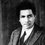# Another divergence proof

Here is another proof for the divergence for the harmonic series . I assume no one has found it earlier! (its awfully dumb to say I'm the first one!!)..... consider the integral $I(a,x) = \int_0^{x} \frac{(\sin x)^{a}}{\cos x} dx$ we will tease this until it cries out an interesting series .....

multiplying 1 continuously OR $(\sin x)^2 + (\cos x)^2$ yields $= \int_0^{x} (\frac{(\sin x)^{a}}{\cos x})((\sin x)^2 + (\cos x)^2) ...... dx$ $= \int_0^{x} (\sin x)^{a}\cos x + (\frac{(\sin x)^{a+2}}{\cos x})((\sin x)^2 + (\cos x)^2) dx$ $= \int_0^{x} ( (\sin x)^{a}\cos x + (\sin x)^{a+2}\cos x + (\sin x)^{a+4}\cos x ....... \infty ) dx$ $= ( \frac{(\sin x)^{a+1}}{a+1} + \frac{(\sin x)^{a+3}}{a+3} + \frac{(\sin x)^{a+5}}{a+5} ..... \infty )$ plug in $a=1 ; x = \frac{\pi}{2}$ $= \frac{1}{a+1} + \frac{1}{a+3} + \frac{1}{a+5} ...... \infty$ to get $= \frac{1}{2} ( \frac{1}{1} + \frac{1}{2} + \frac{1}{3} ...... \infty )$ which is half the harmonic series ........ solving the integral for the same a=1 gives $I(1,\frac{\pi}{2}) = \int_0^{\frac{\pi}{2}} \tan x dx$ $= \infty$ ....... hence the harmonic series diverges!

What is more important here is the behavior of the series as the limit transforms from 0 to x ..... When x starts from 0 further (keeping a as 1 ) and reaches pi/2 the series changes its values according to the function tan x ....... beautiful! isn't it ?Note by Abhinav Raichur
6 years, 1 month ago

This discussion board is a place to discuss our Daily Challenges and the math and science related to those challenges. Explanations are more than just a solution — they should explain the steps and thinking strategies that you used to obtain the solution. Comments should further the discussion of math and science.

When posting on Brilliant:

• Use the emojis to react to an explanation, whether you're congratulating a job well done , or just really confused .
• Ask specific questions about the challenge or the steps in somebody's explanation. Well-posed questions can add a lot to the discussion, but posting "I don't understand!" doesn't help anyone.
• Try to contribute something new to the discussion, whether it is an extension, generalization or other idea related to the challenge.

MarkdownAppears as
*italics* or _italics_ italics
**bold** or __bold__ bold
- bulleted- list
• bulleted
• list
1. numbered2. list
1. numbered
2. list
Note: you must add a full line of space before and after lists for them to show up correctly
paragraph 1paragraph 2

paragraph 1

paragraph 2

[example link](https://brilliant.org)example link
> This is a quote
This is a quote
    # I indented these lines
# 4 spaces, and now they show
# up as a code block.

print "hello world"
# I indented these lines
# 4 spaces, and now they show
# up as a code block.

print "hello world"
MathAppears as
Remember to wrap math in $$ ... $$ or $ ... $ to ensure proper formatting.
2 \times 3 $2 \times 3$
2^{34} $2^{34}$
a_{i-1} $a_{i-1}$
\frac{2}{3} $\frac{2}{3}$
\sqrt{2} $\sqrt{2}$
\sum_{i=1}^3 $\sum_{i=1}^3$
\sin \theta $\sin \theta$
\boxed{123} $\boxed{123}$